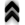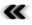### 4.8 Distances

To deﬁne the distance between two objects is a concept universally graspable on an intuitive level. In mathematical terms the notion of distance as reinforced by our every day experiences can be expressed using the simple notion of an aﬃne space (Deﬁnition 79) and the lengths of the vectors inhabiting the vector space, which is part of the aﬃne space’s deﬁnition. This simple notion fails, however, when considering distances between geographical locations, since the shape of the world can not be modelled as an aﬃne space, but necessarily takes the shape of a manifold (Deﬁnition 37), even if this manifold is embeddable into an aﬃne space.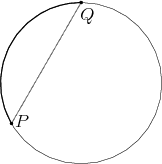Figure 4.7: The distance within the manifold diﬀers from the distance obtained, when it is embedded into an aﬃne space.

A simple example of this circumstance is presented in Figure 4.7. The manifold of a circle is embedded in the aﬃne space of the plane. Considering the simple case, where the circumference of a circle equals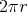, the maximum distance between any two points on the circle is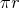, while in contrast the embedded case has a maximum distance of a mere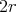.

Therefore a more abstract and general approach is presented in order to alleviate this issue. Before the length can be assessed, it should be noted that the question whether any two points are connected or not, is a question of topology (Deﬁnition 28), not of the geometry built on top of this topology. The connection established by topology manifests itself in the existence of curves (Deﬁnition 45) connecting the two points under consideration. As the number of curves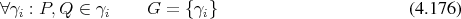joining the points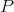andis inﬁnite, even when the set of points involved is unique, since diﬀerent parametrization deﬁne diﬀerent curves, an additional selection criterion, the length of a curve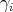, which is independent of the parametrization, is required to select a particular curve and assign the distance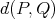. The length of a segment of a curve may be deﬁned using integration (Deﬁnition 95) along the curve.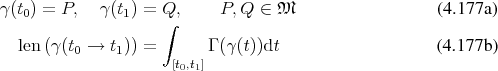The distance is now readily obtained as the result of a minimization of the lengths of all the available curves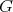as described in Equation 4.176 connecting the desired points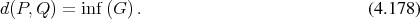As this formulation uses the inﬁmum, it allows for the simple inclusion of open sets, which would otherwise pose a problem. Applying this deﬁnition allows not only for the proper treatment of distances within Riemannian manifolds (Deﬁnition 78), which enable proper treatment of distances along the globe, but also encompasses settings used in the theory of relativity . In these cases the term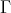is connected to the metric tensor ﬁeld (Deﬁnition 76).